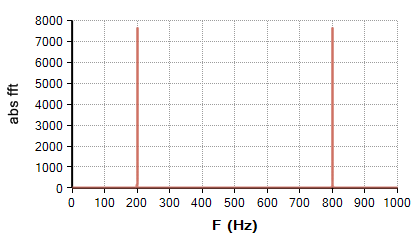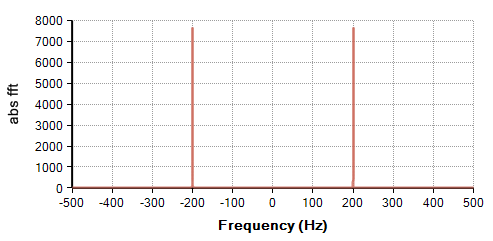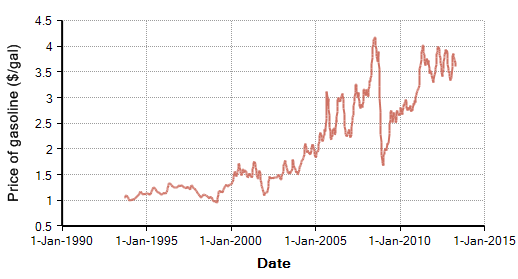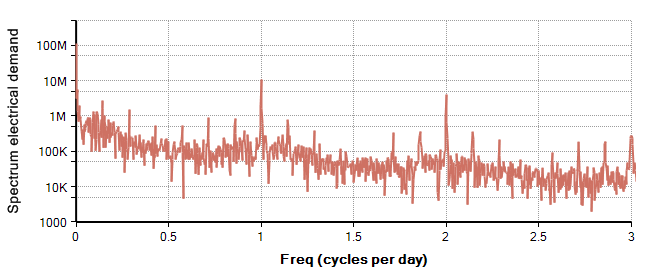# FFT

## FFT(x, T, Freq)

The Fast Fourier Transform (FFT) converts a time series of equally spaced values, «x», from the discrete time (or spatial) domain to the discrete frequency domain. «T» is the time index and «Freq» is the Frequency index, and these two indexes should have the same length. The time series, «x», is indexed by «T» and may be real or complex, and the result is indexed by «Freq» and contains complex numbers in general. The units of «Freq» are cycles per «units of T».

The FFTInv function does the inverse transformation from the frequency domain back to the time (or spatial) domain.

## The Discrete Fourier Transform

The FFT computes the discrete Fourier transform (DFT) in an efficient manner. The DFT is defined given by

$\displaystyle{ H_k = \sum_{i=0}^{n-1} x_i e^{2j\pi i k/n} }$

where $\displaystyle{ j }$ is the imaginary number $\displaystyle{ \sqrt{-1} }$, and n is the number of points in «T» and «Freq». This is a discrete approximation to the continuous Fourier Transform given by

$\displaystyle{ H(f) = \int_{-\infty}^\infty x(t) e^{2j \pi f t} dt }$

### Units and spacing

Let $\displaystyle{ \Delta t }$ denote the spacing between points in «T» and $\displaystyle{ n }$ be the number of points. Then the spacing $\displaystyle{ \Delta F }$ of the points in «Freq» is $\displaystyle{ 1/n\Delta t }$. The quantity $\displaystyle{ 1/\Delta t }$ is called the sampling frequency, which should be at least twice the smallest frequency that is present in the underlying continuous signal.

For example, suppose your time series is sampled at 1000Hz for just over 10 seconds, so that $\displaystyle{ \Delta t=1 millisecond }$ and $\displaystyle{ n=10K }$. Then $\displaystyle{ \Delta F=0.1 Hz }$. It would make sense to define «Freq» as (0..10K-1)*0.1 so that the numeric values of «Freq» are in Hertz.

### Nyquist frequency, aliasing, mirroring

When all frequencies that are present in the underlying continuous signal are below the Nyquist frequency, $\displaystyle{ 1/2\Delta t }$, then the discretely sampled time series contains all of the information in the original continuous signal. This is known as the sampling theorem and is a remarkable fact. Even though the continuous signal appears to contain infinitely more information -- all the values between the discrete points in time where the signal is sampled -- the discrete series uniquely determines the full continuous series.

When a frequency is present that exceeds the Nyquist frequency, the power from that frequency is still transferred to the FFT result, but it gets mapped to a bin in the result as if it had wrapped around. This phenomena is termed aliasing. So you can think of the resulting spectrum as being a circular buffer, representing signal at each frequency modulo the maximum frequency of $\displaystyle{ 1/\Delta t }$. A frequency of $\displaystyle{ 1/2 \Delta t }$ would map to the middle bin, but so would a frequency of $\displaystyle{ 3/2\Delta t }$ as well as a frequency of $\displaystyle{ 5/2\Delta t }$.

The same wrap-around occurs for negative frequencies. When the real-valued time series contains a component sine wave with a frequency of 100 Hz, it implicitly also contains a frequency of -100Hz. This -100Hz component also appears in the result of the FFT, but instead of mapping to a negative bin, it wraps around and appears in the second half the the spectrum. Hence, the result of FFT can be divided into a first half and a second half. For a band-limited signal with all frequencies below the Nyquist frequency, the first half of the spectrum corresponds to positive frequencies, the second half of the spectrum is the negative frequencies.

For example, here is the magnitude of an FFT for a 200 Hz sine wave, Sin(200*T*360) where T is in secondsIn the example, $\displaystyle{ \Delta t }$ is 1 ms, so the maximum frequency is 1KHz. Notice that the 200Hz signal shows up both at 200Hz and at 800Hz -- the 800 Hz actually capturing the -200Hz component.

In many applications, an FFT is taken, the signal is multiplied by a transfer function and the inverse FFT is then applied. In these cases, it is usually most convenient to keep the frequency spectrum in this wrapped-around representation. But when displaying a spectrum, you might want to either extract only the first half the array, thus showing only the positive frequencies, or remap it to a frequency index that has negative values. In either case, you'll need a second frequency index.

Suppose your F index is (0..10K-1)*0.1. To extract the positive part:

Index Freq_pos := (0..5K-1) * 0.1 Do fft_result[Freq=Freq_pos]

To map to an index with a negative part:

Index Frequency := (-5K + 1..5K)*0.1 Do fft_result[@Freq = Mod(@Frequency + 5K - 1, 10K) + 1]

The use of positional indexing here avoids potential complications due to numeric round-off.### Amplitude and phase

Each number in the result of FFT is a complex number. You can think of this as an encoding of both the amplitude and phase of each frequency component. For example, if a 200 Hz component is present, the magnitude of the result at 200Hz (given by the Abs function) gives the power density at that frequency. But if you are to recover the original signal, the phase of the component is also relevant. Even though the power density at 200Hz is the same for a Sin(200*T*360), a Cos(200*T*360), or a Sin(200*T*360 + 30), the phase is different in each of these cases. The ComplexDegrees or ComplexRadians function can be used to extract the relative phase of each component.

I always find the absolute height of an FFT to be somewhat confusing. What is usually important is the relative height across different frequencies -- e.g., there 1000 more power at 200Hz than at 100Hz. The height is a reflection of power density, so if you double the sampling frequency, and hence half the width of each frequency bin, you'll double the amplitude of the FFT result.

## Efficiency

The FFT is a fast implementation of the Discrete Fourier Transform (DFT), which is most efficient when the number of elements in «T» and «Freq» is an even power of 2. Analytica's implementation is still considerably more efficient than a straight DFT as long as the number of elements in «T» is the product of several small factors. So, for example, it would still be very efficient when «T» has 531,441 items, since $\displaystyle{ 531441=3^{12} }$. It is also pretty good on even powers of 10, since even powers of 10 have factors of 2 and 5, both of which are small numbers. The worst efficiency would occur when «T» has a prime number of elements, in which case the FFT would be equivalent in efficiency to a DFT and would have $\displaystyle{ O(n^2) }$ time complexity.

A straight DFT requires $\displaystyle{ O(n^2) }$ steps. If you were to apply this to a time series having n=1M points, it would require 1 trillion steps to compute, which is likely to be infeasible. The complexity of an FFT when the number of points is an even power of 2 is computed in $\displaystyle{ O(n \log(n)) }$ steps. For n=1M this comes out to 6 million steps, about 150,000 times faster than a straight DFT.

I haven't worked out the complexity for the case when n is not a power of 2, but is a product of small factors, but I think it should be something like $\displaystyle{ O(k n \log(n)) }$, where k is the largest factor and k « n.

## Examples

One application of the FFT is to detect (and remove) cyclic or seasonal components in data before applying regression techniques to fit forecasting models to the data. You plot the absolute value of the FFT for your data and see if any dominant frequencies stand out -- these would correspond to seasonalities. These are usually spotted by human eye, and then removed.

To demonstrate, here is a graph of average weekly gasoline price at the pump in the US over the previous 1024 weeks (from U.S. Energy Administration Information):Each of the 1024 data points is spaced 7 days apart, so we define the «Freq» index to be (0..1023) / (1024*7)*365.25. The last 365.25 factor is to put the units in cycles per year, so Freq = 1 would be the annual cycle, and Freq = 12 would be a monthly seasonality.

Abs(FFT(Price_of_gasoline, Date1, Freq) →Surprisingly, this is an example where no seasonal component is evident. Pure white noise has a flat spectrum. Even though this graph is shown on a log scale, it is quite flat.

In another example, we look at the frequency spectrum for hourly electrical demand in New England from 1-Jan-2011 to 31-Oct-2011. Here the time-series data is on the Hour index, and we define the Freq index as (0..size(hour)-1)*1/(size(hour))*24. The 24 here is to convert frequency units to cycles per day instead of cycles per hour. «Freq» ranges from 0 to 24, with the following graph manually scaled to the section showing frequencies under 3 cycles per day.In this case two cyclic components are evident a daily cycle (at Freq = 1) and a 12 hour cycle (at Freq = 2). Before applying regression techniques, these two peaks could be removed. Instead of setting the peaks to zero, I'm going to reduce the peaks to be similar in amplitude to the neighboring data points. By looking at the table view, we find the peaks to be about 3 cells wide (although the middle cells is dominant) so I will adjust a few neighboring cells. The adjusted_spectrum is:

 Var h := FFT(Electricity_demand, Hour, Freq);
Var adjust := If 0.995 < Freq < 1.005 Then 550K/Abs(h)
Else If 1.995 < Freq < 2.005 Then 80K/Abs(h)
Else 1;


and the time series with this seasonality removed is given by

Abs(FFTInv(adjusted_spectrum, Freq, Hour))

We estimated the 550K and 80K target levels for Freq = 1 and Freq = 2 by looking at the neighboring cells. In this example, the seasonally adjusted time series is nearly indistinguishable from the original (since the seasonality is so small), but the steps at least demonstrate the technique.

### Fast convolution

Another application of the FFT is fast convolution. Convolution itself has several uses. It can be used to average neighboring values together to smooth out noise, or conversely to enhance change points. It is also used to a transfer function describing how one component acts on a signal. When computed in the time domain, convolution requires $\displaystyle{ O(n^2) }$ steps, whereas it becomes a simple multiplication in the frequency domain. Hence, it is actually more efficient to use the FFT to transform a time series and a filter shape or transfer function into the frequency domain, multiply their spectra together, and then use FFTInv to transform the result back to the time domain.

### Characteristic functions of probability distributions

The FFT can be useful for computing a full probability density curve for the distribution of a sum of independent random variables. Suppose x1, x2, ... xn are each random variables with distributions p1(x1), p2(x2),..., pn(xn), and let p(x) be the distribution of x=x1+x2+...+xn. Then to within a constant, k,

$\displaystyle{ FFT(p) = k \sum_{i=1}^n FFT(p_i) }$

This means that in many cases, the full distribution curve for a sum of random variables can be quickly computed by way of the FFT.

The FFT is closely related to the characteristic function of a probability distribution p(x), which is defined as:

$\displaystyle{ CF(\omega) = E_p[e^{i \omega x}] = \int_{-\infty}^\infty p(x) e^{i \omega x} dx }$

Comparing $\displaystyle{ CF(\omega) }$ to the Fourier transform, H(p), you can see that the CF is simply the FFT with a change of units -- radians instead of cycles.

The application of the FFT to computing the probability curve involves a trade-off between the number of points to use and what range of values to span. When your distributions have long tails, as you cover more of the tail, you get sparser coverage of the area around the mode.

## History

Introduced in Analytica 4.5.